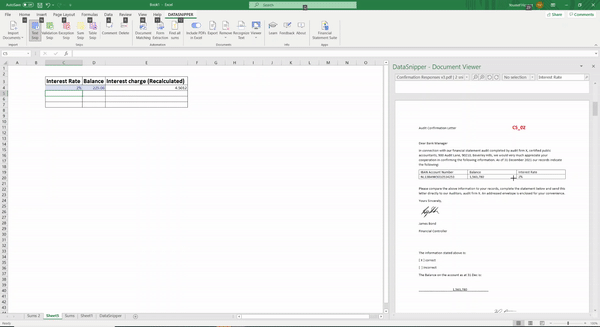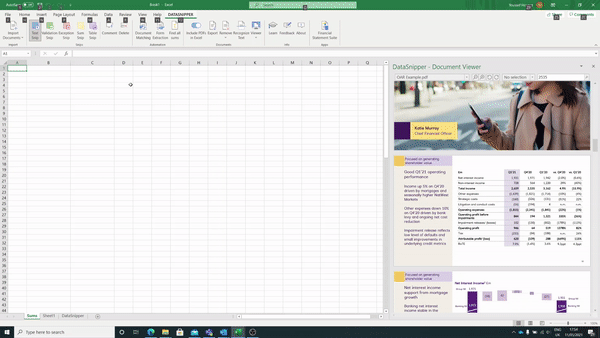# How to perform recalculation procedures using DataSnipper

## Learn how to use DataSnipper to perform recalculation procedures, you can use the sum snip, text snip or combinations to recalculate balances within client reports.

### Recalculating totals using DataSnipper

You can instantly recalculate totals within a client's document or a piece of evidence using the sum snip within DataSnipper. Simply highlight the specific balances you would like to add together and click and drag across them to recalculate the overall total of these balances.

### Extract specific figures and recalculate formula using DataSnipper

For more complex calculations you can use the 'text snip' to extract specific terms and then use these figures to recalculate the sample item. As an example if you have an interest rate and a balance you can extract both using the text snip and then multiply these together to recalculate the interest charge.### Find all sums

You can also use the 'Find all Sums' functionality within DataSnipper to perform recalculation procedures over multiple totals at once. Simply import the specific report you would recalculate totals of and select the relevant button in the automation tab.

DataSnipper creates a new sheet and adds a Text Snip from the totals of a sum. Column B will be filled with the totals of a Sum and column C with the parts. You can easily review the mathematical accuracy by scrolling through the documents of the snips.